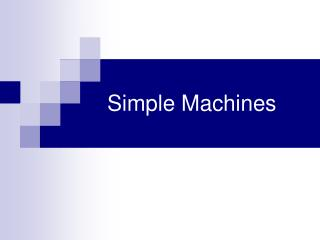# Simple Machines - PowerPoint PPT PresentationDownload PresentationSimple Machines

Simple MachinesDownload Presentation## Simple Machines

- - - - - - - - - - - - - - - - - - - - - - - - - - - E N D - - - - - - - - - - - - - - - - - - - - - - - - - - -
##### Presentation Transcript

1. Simple Machines

2. Simple Machines make work easier for us. • What is work? • Work occurs when a force causes an object to move in the direction of the force.

3. This means that if you are simply holding a heavy object, you are not doing any work. • Even if an object is moving, work is only done if the force is in the direction of motion.

4. No work is being done on the suitecase.

5. The formula to calculate work:WORK = FORCE x DISTANCE Units of measurement:Force – newtonsDistance – metersWork – newton-meter = joule

6. Power Is a measure of how fast work is done.POWER = WORK TIME Units of measurement:Work – joulesTime – secondsPower – joules/second = watt

7. Simple Machines • Machines are devices that make work easier by changing the SIZE or DIRECTION of a FORCE. • Machines do not reduce the amount of work for us, but they can make it easier.

8. The Force-Distance Trade-off • A machine can reduce either the FORCE or DISTANCE required to do work, but not both. • If FORCE is DECREASED, the DISTANCE the force is exerted must INCREASE.

9. Six types of Simple Machines • Inclined Plane • Lever • Wheel & Axle • Pulley • Screw • Wedge

10. Examples

11. More Examples

12. ORGANIZER

13. ORGANIZER

14. Levers • A lever is a simple machine made with a bar free to move about a fixed point called a fulcrum. There are three types of levers.

15. LOAD LOAD Input Force Output Force • A first class lever has the fulcrum between the INPUT FORCE and the OUTPUT FORCE. Fulcrum

16. Examples:

17. LOAD Input Force • In a second class lever, the LOAD is between the FULCRUM and the INPUT FORCE. Fulcrum

18. Examples of Second Class Levers

19. LOAD • In a third class lever, the INPUT FORCE is between the FULCRUM and the LOAD. Output Force Fulcrum Input Force

20. Example of a Third Class Lever

21. FULCRUM LOAD IGNORE THE “A” EFFORT FORCE F L E

22. 2nd 3rd 1st F L E

23. LOAD 1st class lever: the FULCRUM is between the LOAD and the EFFORT FORCE 1st Effort Force F L E Fulcrum

24. LOAD 2nd class lever: the LOAD is between the FULCRUM and the EFFORT FORCE 2nd F L E Fulcrum Effort Force

25. LOAD 3rd class lever: the EFFORT FORCE is between the FULCRUM and the LOAD 3rd F L E Fulcrum Effort Force

26. Pulleys • A pulley is a simple machine made with a rope, belt or chain wrapped around a grooved wheel.

27. A movable pulley is attached to the object you are moving. • A fixed pulley changes the direction of the applied force. ( Ex. Raising the flag ) .

28. Inclined Plane • An inclined plane is a simple machine with no moving parts. • It is simply a straight slanted surface. example: a ramp

29. Wheel and Axle • The wheel and axle must move together to be a simple machine. • Sometimes the wheel has a crank or handle on it. • Examples: roller skates and doorknobs.

30. Wedge • A WEDGE is made of two inclined planes put together. • Example: A knife blade

31. Screw • A screw is an inclined plane that is wrapped around a shaft. • Example: Bottle cap

32. Mechanical Advantage OUTPUT FORCE • Mechanical advantage tells you how many times a machine multiplies force. = Mechanical Advantage INPUT FORCE (Input force means the same as effort force)

33. If a lever has a MECHANICAL ADVANTAGE of 5, the output force is 5 times the input force. • Therefore, the INPUT FORCE must travel 5 times the distance of the OUTPUT FORCE

34. load We can calculate the mechanical advantage of a lever by comparing the effort arm to the load arm. Length of Effort Arm MA lever = Length of Load Arm

35. The Load Arm is often called the Resistance Arm

36. LOAD Lever with Mechanical Advantage of 1 Length of Effort Arm = Length of Load Arm

37. LOAD A Mechanical Advantage of 1 means that the OUTPUT FORCE is the same as the INPUT FORCE. Input Force Why would you want this type of lever? Output Force

38. LOAD 5 times Distance AB Distance BC Lever with Mechanical Advantage of 5 C B A =

39. LOAD A Mechanical Advantage of 5 means that the OUTPUT FORCE is 5 times the INPUT FORCE. Input Force The input force must move through a distance 5 times greater than the distance the load moves. Output Force

40. load We can calculate the mechanical advantage of an inclined plane by comparing the length of the plane to the distance the load is lifted. Length of Plane MA inclined plane = Height the load is lifted

41. load Example: 8 feet 2 feet 8 feet 4 MA inclined plane = = 2 feet

42. Summary • Simple machines are "simple" because most have only one moving part. • Machines do not reduce the amount of work for us, but they can make it easier. • "Work" is only done when something is moved in the direction of a force. • Work is the product of effort and distance.